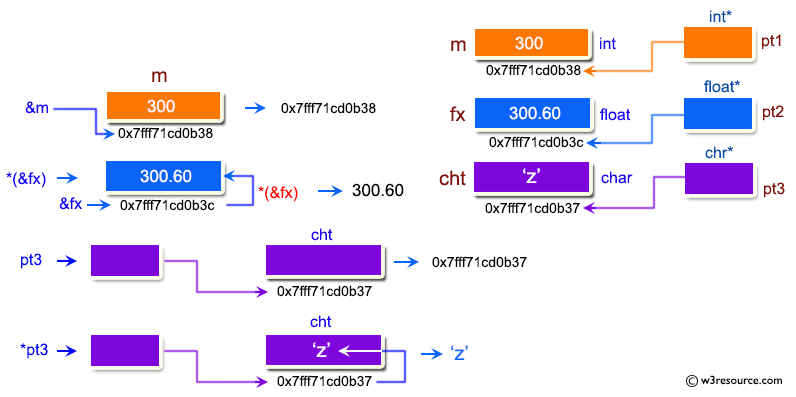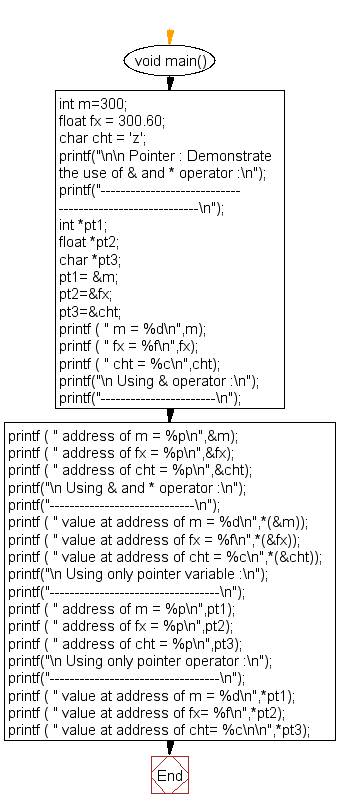﻿ C Program: Demonstrate the use of & and * operator - w3resource# C Exercises: Demonstrate the use of & and * operator

## C Pointer : Exercise-3 with Solution

Write a program in C to demonstrate the use of &(address of) and *(value at address) operator.

Pictorial Presentation:Sample Solution:

C Code:

``````#include <stdio.h>
void main()
{
int m=300;
float fx = 300.60;
char cht = 'z';

printf("\n\n Pointer : Demonstrate the use of & and * operator :\n");
printf("--------------------------------------------------------\n");

int *pt1;
float *pt2;
char *pt3;
pt1= &m;
pt2=&fx;
pt3=&cht;
printf ( " m = %d\n",m);
printf ( " fx = %f\n",fx);
printf ( " cht = %c\n",cht);
printf("\n Using & operator :\n");
printf("-----------------------\n");
printf ( " address of m = %p\n",&m);
printf ( " address of fx = %p\n",&fx);
printf ( " address of cht = %p\n",&cht);
printf("\n Using & and * operator :\n");
printf("-----------------------------\n");
printf ( " value at address of m = %d\n",*(&m));
printf ( " value at address of fx = %f\n",*(&fx));
printf ( " value at address of cht = %c\n",*(&cht));
printf("\n Using only pointer variable :\n");
printf("----------------------------------\n");
printf ( " address of m = %p\n",pt1);
printf ( " address of fx = %p\n",pt2);
printf ( " address of cht = %p\n",pt3);
printf("\n Using only pointer operator :\n");
printf("----------------------------------\n");
printf ( " value at address of m = %d\n",*pt1);
printf ( " value at address of fx= %f\n",*pt2);
printf ( " value at address of cht= %c\n\n",*pt3);
}
``````
``` ```

Sample Output:

```Pointer : Demonstrate the use of & and * operator :
--------------------------------------------------------
m = 300
fx = 300.600006
cht = z

Using & operator :
-----------------------

Using & and * operator :
-----------------------------
value at address of m = 300
value at address of fx = 300.600006
value at address of cht = z

Using only pointer variable :
----------------------------------

Using only pointer operator :
----------------------------------
value at address of m = 300
value at address of fx= 300.600006
value at address of cht= z
```

Flowchart:C Programming Code Editor:

Have another way to solve this solution? Contribute your code (and comments) through Disqus.

What is the difficulty level of this exercise?

Test your Programming skills with w3resource's quiz.

﻿

## C Programming: Tips of the Day

How to format strings using printf() to get equal length in the output?

You can specify a width on string fields, e.g.

`printf("%-20s", "initialization..."); `

And then whatever's printed with that field will be blank-padded to the width you indicate.

The - left-justifies your text in that field.

Ref : https://bit.ly/34DMOc3